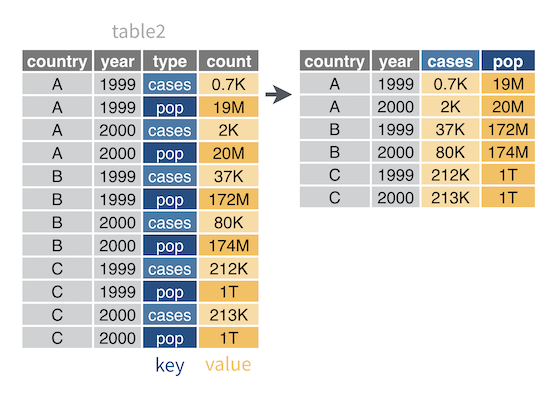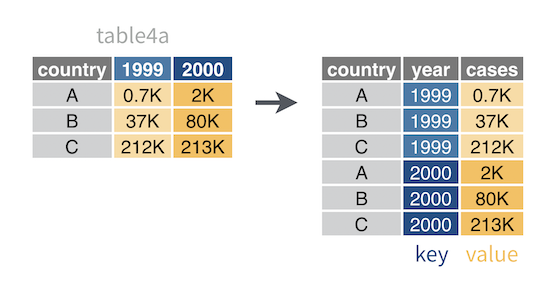``````#clean environment
remove(list = ls())

#check working directory
getwd() ``````
``##  "/Users/naominewmacbook/Desktop/Post-Doc I/R Tutorial"``
``````#load in data

#load packages (we won't need all of them today, but it's good practice to always begin by loading in some frequently used packages)
library(lme4)
library(lmerTest)
library(dplyr)
library(plyr)
library(ggplot2)
library(effects)
library(png)
library(tidyverse)

#tell R not to use scientific notation
options(scipen=999)``````

# Statistical Tests and Modelling in R

##1. Figure out how your data is organized R is extremely powerful, which makes it possible to run statistical analysis on large data sets very quickly. However, before you can start running tests, it is critical to ensure that your data is properly cleaned and in the right format. Otherwise, R might apply built-in settings to deal with NAs, duplicates, etc. and you might end up with inaccurate output.

Generally, data is organized into long or wide format and different types of tests require different formats. When your data is non in the right format to run the desired statistics, you have to reformat (i.e., transform) your columns of interest.

In Sessions 2 and 3, we covered a form of data transformation that involved using calculations for descriptive statistics (ddply summarise commands). To run statistical tests, you will have to transform raw data, although the unerlying logic is similar. Tip: Before you begin running code to transform your data, write out what you want it to look like on a piece of paper (i.e., how should your groups, dependent and independent variables be organizes)

Let’s start by creating some fake data in long format

``````country <- c("A","A","A","A", "B","B","B","B", "C", "C", "C", "C")
year <- c(1999,1999, 2000, 2000, 1999,1999, 2000, 2000, 1999,1999, 2000, 2000)
type <- c("cases", "pop", "cases", "pop", "cases", "pop", "cases", "pop", "cases", "pop", "cases", "pop")
count <- c(0.7,19,2,20,37,172,80,174,212,1000,213,1000)

DF1 <- data.frame(country, year, type, count)``````

and some more fake data in wide format

``````country <- c("A", "B", "C")
"1999" <- c(0.7, 37, 212)
"2000" <- c(2, 80, 213)

DF2 <- data.frame(country, 1999, 2000)``````

A. Spread: Most of the programs we use in Psycholinguistics produce trial level output, which is generally considered long format data. We can use spread() to transorm it to wide format. This means spreading a pair of columns (one coding “key” and another coding “value”) into a field of cells. It will move variable names out of the cells and into the column names.

Here’s a visualization of how what data transformation from long to wide format looks like:let’s try it

``````wideDF <- DF1 %>%
spread(key = type, value = count)``````

B. Gather: The opposite of spread() data is gather(). This is used when you want to convert wide data to long, reshape a two-by-two table, or move variable values out of the column names and into the cells. Note that when you gather data, you are creating new columns. So you will have to pick names for the new key and value columns, and supply them as strings in the command. Then identify the columns to gather into the new key and value columns.

Here’s a visualization of how what data transformation from wide to long format looks like:## 2. Subset as needed

We will return to using the “WordImageMatching_Tutorial_May2021” that we loaded in at the top. We are interested in analyzing correct reaction times, so we will create a subset that only contains observations for trials that were responded to correctly

``correct <- subset(WordImageMatching_Tutorial_May2021, Accuracy == 1)``

##3. Check distribution of the DV The test we will be covering today assume normal distribution of the DV. We can check this using a simple histogram of our DV (in this case reaction time).

``hist(correct\$ReactionTime)``